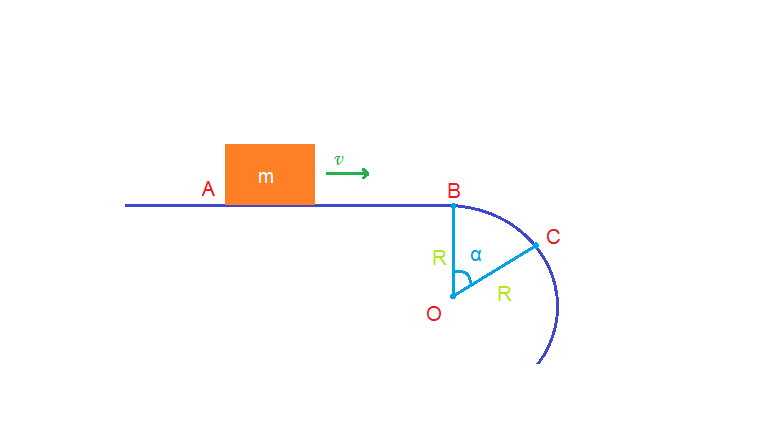Block sliding off a tableA small block slides on a horizontal friction-less surface $AB$ of a table with speed $\frac { 1 }{ 2 } \sqrt { gR }$. At some point $B$, the track becomes circular with radius $R$ (look at the diagram). The block leaves the table at a certain point $C$. Determine the angle $\alpha$.

×